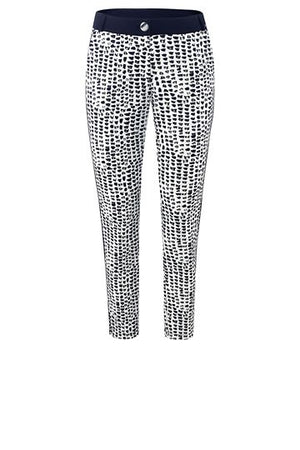Airfield

# Black & White Print Pant

Regular price \$435 now \$130.50 Unit price  per

Shipping calculated at checkout.
• 94% polyester 6% elasthan
• Machine wash cold
• Hang to dry
• Scuba fabric
• Straight leg
• Fits slim
• Mid rise
• Slit on ankle

Size Guide

• 34 = 4 = XS
• 36 = 6 = S
• 38 = 8 = M
• 40 = 10 = M
• 42 = 12 = L
• 44 = 14 = L
• 46 = 16 = XL